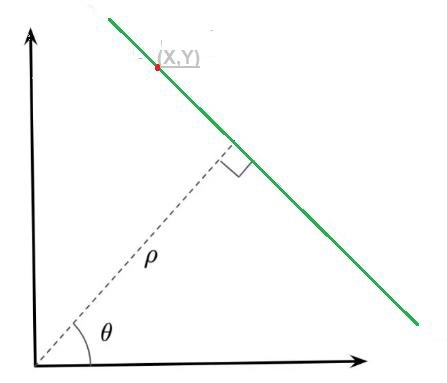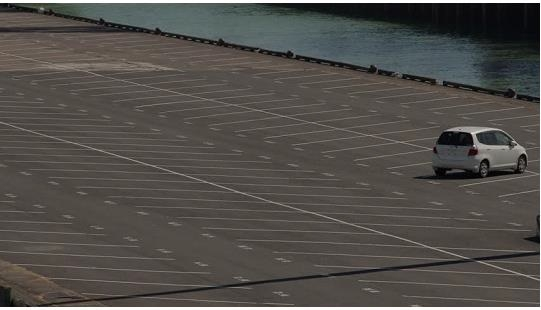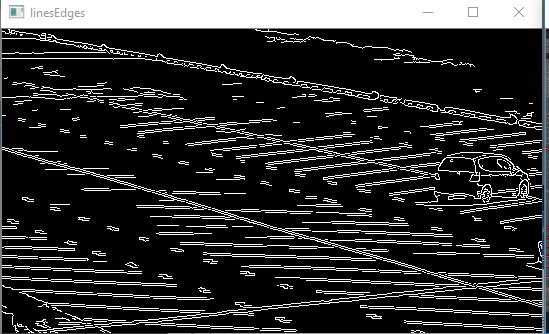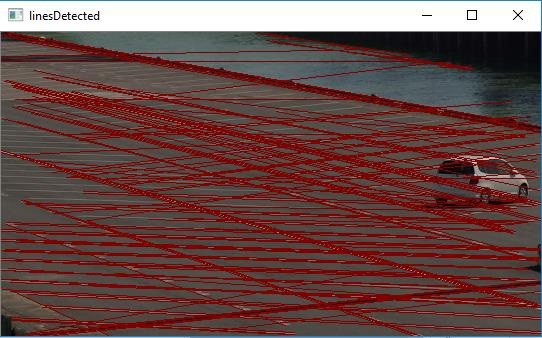# Line detection in python with OpenCV?

In this post, we are going to learn, how to detect lines in an image, with the help of a technique called Hough transform.

## Hough transform?

Hough transform is a feature extraction method to detect any simple shape, if you can represent that shape in mathematical form. It somehow manage to detect the shape even if it is broken or distorted a little bit. We will see how it works for a line.

A “simple” shape is one that can be represented by only a few parameters. For example, a line can be represented by two parameters only (slope, intercept) and a circle has three parameters – the coordinates of the center and the radius (x,y, r).

## Detect lines from an image using Hough transform

A line can be represented by an equation-  or in parametric form it can be representated as, as  where (ρ) is the perpendicular distance from origin to the line, and ϴ is the angle formed by this perpendicular line and horizontal axis measured in counter-clockwise (This representation is used in OpenCV). Check below imageSo if line is passing below the origin, it will have a positive rho and angle less than 180. If it is going above the origin, instead of taking angle greater than 180, angle is taken less than 180, and rho is taken negative. Any vertical line will have 0 degree and horizontal lines will have 90 degree.

## Accumulator

Any line can be representated in these two terms (ρ,ϴ). So first it creates a 2D array or accumulator (to hold values of two parameters) and it is set to 0 initially. Where rows denote the ρ and columns denote the ϴ. Size of array depends on the accuracy we need, for example, if we need accuracy of angles to be 1 degree, you need 180 columns and ρ, is the maximum distance possible is the diagonal length of the image and ρ is the maximum distance possible is the diagonal length of the image. So taking one pixel accuracy and number of rows can be diagonal length of the image.

Consider we have an image of 100*100 with a horizontal line at the middle. Take the first point of the line, and we know its (x,y) values. Now in the equation, pu the values ϴ=0,1,2,3…180 and check the ρ value you get. For every (ρ,ϴ) pair, increment value by one is our accumulator in its corresponding (ρ,ϴ) cells. So now in accumulator, the cell (50,90) = 1 along with some other cells. Now take the second spot on the line. Repeat the same procedure as we did for first spot. Increment the values in the cells corresponding to (ρ,ϴ) you get. This time, the cell (50,90)=2. So we are actually voting the (ρ,ϴ) values. We continue this process for every point on the line. At each spot, the cell (50,90) will be incremented or voted up, while other cells may not be voted up. This way, at the end, the cell(50,90) will have maximum votes. So if you search the accumulator for maximum votes, you get the value (50,90) which says, there is a line in this image at distance 50 from origin and at angle 90 degrees. This is how hough transform for lines works.

## Hough Transform in OpenCV

Everything explained above is encapsulated in the OpenCV function, cv2.HoughLines(). It simply returns an array of (ρ,ϴ) values where ρ is measured in pixels and ϴ is measured in radians.

Below is a program of line detection using openCV and hough line transform.

Below is actual image of a parking lot, and we are going to do line detection on this image using hough line transform and OpenCV library.## Example

import cv2
import numpy as np
gray = cv2.cvtColor(img, cv2.COLOR_BGR2GRAY)
edges = cv2.Canny(gray, 75, 150)
lines = cv2.HoughLinesP(edges, 1, np.pi/180, 30, maxLineGap=250)
for line in lines:
x1, y1, x2, y2 = line
cv2.line(img, (x1, y1), (x2, y2), (0, 0, 128), 1)
cv2.imshow("linesEdges", edges)
cv2.imshow("linesDetected", img)
cv2.waitKey(0)
cv2.destroyAllWindows()

## ResultAnd lines detected−